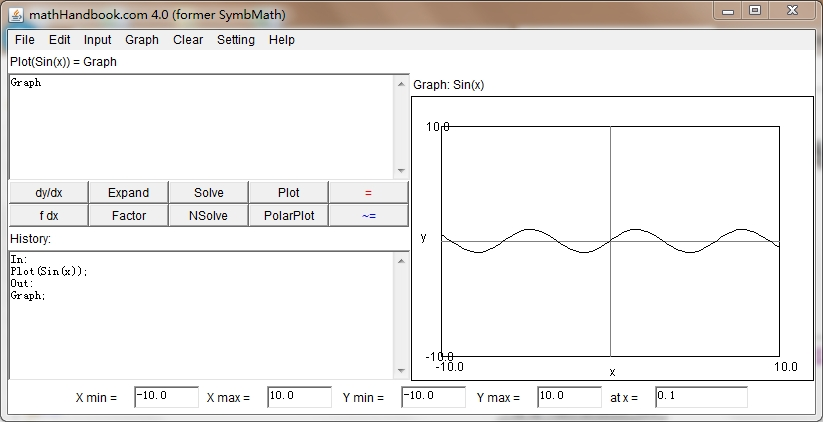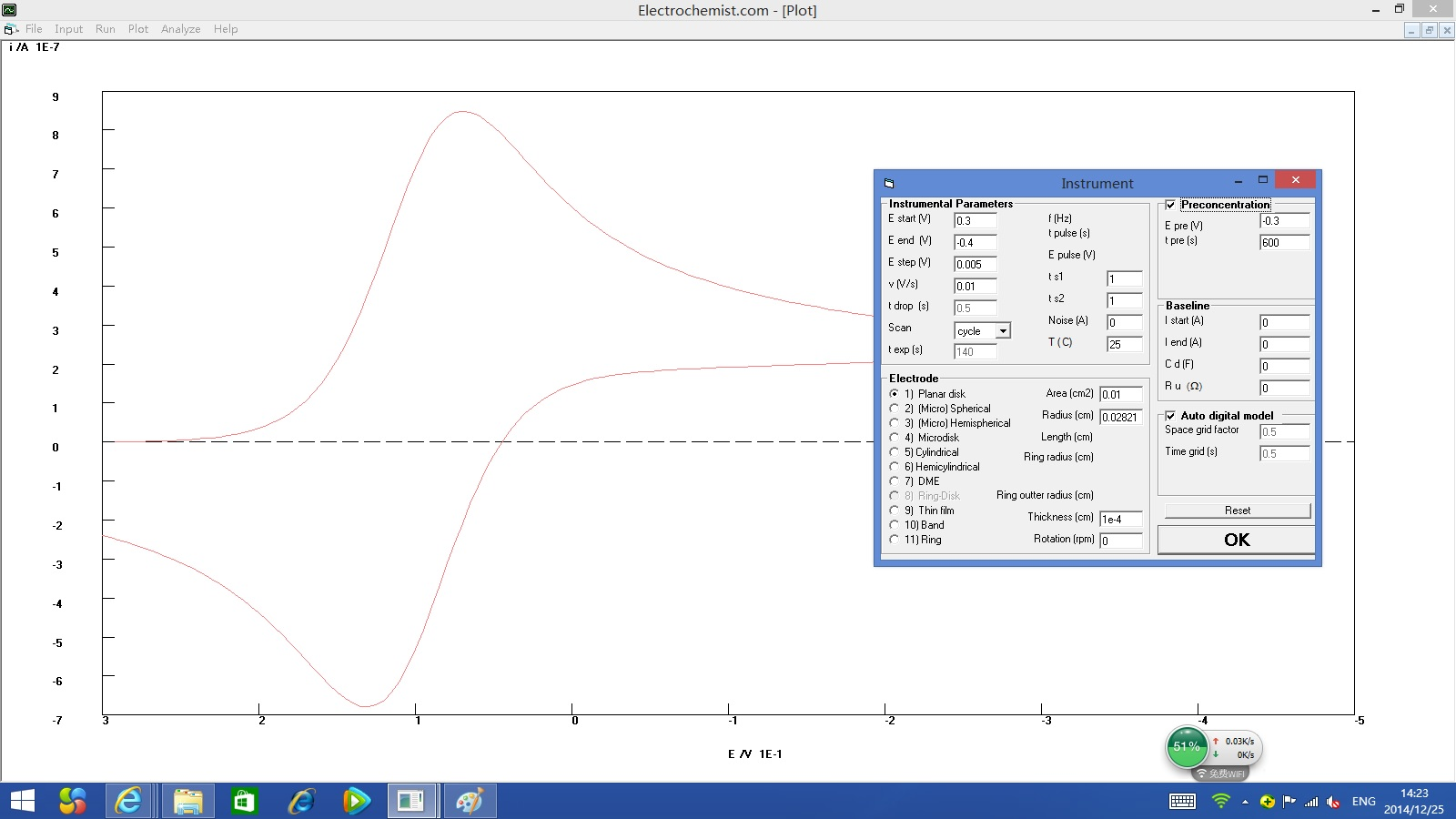﻿ ﻿﻿ Fractional Calculus Computer Algebra System math software Home | list | math | function | calculator | handbook | help | forum | contact | index | 中文﻿

# Fractional Differential Equation

﻿
1. ## Differential equation

By default, the unknown function is y(x) and its variable is x and its initial value is y(0).
there are 3 way to input derivative y: y', y(1,x), ds(y,x), d(y(x))
there are 3 way to input second order derivative y: y'', y(2,x), ds(y,x,2), d(y(x),x,2)
there are 2 way to input the 0.5 order derivative y: y(0.5,x), ds(y,x,0.5), d(y(x),x,0.5)
Input y(1,x) as first order differential equation dy/dx - 2y=0

2. ## Fractional differential equation

Differential equation is extend to fractional differential equation by fractional order. e.g. input ds(y,x,0.5) as semi differential equation d^0.5/dx^0.5 y = 2y by fractional calculus.

3. ## Complex order of differential equation

Differential equation is extend to complex order of differential equation by extension of order to complex number. e.g. input ds(y,x,i) as complex order of differential equation d^(i)/dx^(i) y = 2y by fractional calculus.

4. ## Fractional integral equation

Integral equation is extend to fractional integral equation by minus fractional order. e.g. input ds(y,x, -0.5) as semi integral equation d^-0.5/dx^-0.5 y = 2y

5. ## Solve (fractional) differential equation

Property of a fractional differential equation is the same as a differential equation.
Solution of linear fractional differential equation = general solution + parcular solution = gsolution()+psolution(),
similar to linear differential equation. So method to solve fractional differential equation is similar to differential equation.

Integral equation can be converted to differential equation by differentiating both sides. Some fractional integral equation aslo can be converted to fractional differential equation, but not every fractional integral equation can.

Solve (fractional) differential equation for y by dsolve() or lasolve(), e.g.
dsolve( y'=2y )
dsolve( d^0.5/dx^0.5 y=2y )
lasolve( d^0.5/dx^0.5 y=2y )

By default, the Caputo definition is used by dsolve(). If you want to use the Riemann defintion, use the Laplace transform solver lasolve().
lasolve(y(-0.5,x)=1) give nonzero.
dsolve(y(-0.5,x)=1) give zero.

6. ## Solve system of differential equations

By default, the unknown function are x(t) and y(t) and their variable is t, their initial value are x(0) and y(0).
dsolve(x(1,t)=x-t,y(1,t)=t-x)
x(0):=1, y(0):=1, lasolve(x(1,t)=x-t,y(1,t)=t-x)

7. ## Test solution for (fractional) differential equation

The odetest() or test() is the ode test to test solution for (fractional) differential equation, e.g.
test( exp(4x), d^0.5/dx^0.5 y=2y )
f:= 2y+1, eq:=y'-f=0, s:=dsolve(eq), test(s, eq)
f:= 2y+1, eq:=ds(y,x,0.5)-f=0, s:=dsolve(eq), test(s, eq)

8. ## Comparison of differential equations

Ordernameequationgeneral solutionparcular solution
icomplex order differential equation d^i/dx^i y - 2y=exp(x)C_1*exp(1/2^i*x)-exp(x)
2second order differential equation d^2/dx^2 y - 2y=exp(x)C_1*exp(sqrt(2)*x)-exp(x)
1.51.5 order differential equation d^1.5/dx^1.5 y - 2y=exp(x)C_1*exp(2^(2/3)*x)-exp(x)
1 differential equation d/dx y -2y=exp(x)C_1*exp(2*x)-exp(x)
0.5semi differential equation d^0.5/dx^0.5 y - 2y=exp(x)C_1*exp(4*x)-exp(x)
-0.5semi integral equation d^-0.5/dx^-0.5 y - 2y=exp(x)C_1*exp(1/4*x)-exp(x)
-1integral equation int y\ dx-2y=exp(x)C_1*exp(1/2*x)-exp(x)
solution= general solution+parcular solution = gsolution()+psolution()

All of their solutions are in the same format exp(k x). When the n order of the differential equation decreased from 2 to 0.5, its general solution increased from exp(sqrt(2)*x) to exp(4x). When the n order of the differential equation decreased from -0.5 to -1, its general solution increased from exp(1/4 x) to exp(1/2 x). but their parcular solutions are unchanged. Their change are similar to a change of the n order in fractional derivative d^n/dx^n x in below picture.

﻿
See Also

Copyright 2018 DrHuang.com